# Playground

The land for the school playground's construction has the shape of a rectangle with a shorter side of 370 m. Its other side is 260 m longer. How many meters of the fence does the school need to buy on the fencing playground?

x =  2000 m

### Step-by-step explanation: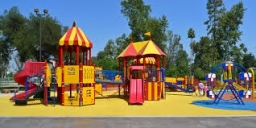Did you find an error or inaccuracy? Feel free to write us. Thank you!#### You need to know the following knowledge to solve this word math problem:

We encourage you to watch this tutorial video on this math problem:

## Related math problems and questions:

• The playgroundThe playground has the shape of a square with a side of 64 m. It is fenced on three sides. What is the area of the playground and how long is its fence?
• Playground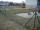Fencing square playground cost € 464; 1-meter cost € 19. What is the area of the playground?
• Fence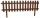How many meters of fencing it is required to purchase if our garden is rectangular measuring 20 m and 180 dm.
• Two sides paintThe door has the shape of a rectangle with dimensions of 260cm and 170cm. How many cans of paint will be needed to paint this door if one can of paint cover 2m2 of the area? We paint the doors on both sides.
• Rectangle - sidesWhat is the perimeter of a rectangle with area 266 cm2 if length of the shorter side is 5 cm shorter than the length of the longer side?
• Fence of the garden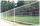The garden has dimensions of 5m and 400cm. How many meters meshes are needed for fencing the plot?
• Wire fenceThe wire fence around the garden is 160 m long. One side of the garden is three times longer than the other. How many meters do the individual sides of the garden measure?
• LandRectangular triangular land has area 30 square meters and 12 meters long leg. How many meters of the fence do you need for fencing this land?
• Two lands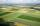The common area of the two neighboring lands is 964 m2. The second land is 77 m2 smaller than twice the size of the first land. Find the areas of each land.
• GardenThe area of a square garden is 2/9 of triangle garden with sides 160 m, 100 m, and 100 m. How many meters of fencing need to fence a square garden?
• Horses playgroundThe fence for the horses has the shape of a rectangular trapezoid with an area of 400 m2, the base lengths should be 31 m and 19 m. How many meters of boards will they need to fence it if the boards are stacked in 5 rows?
• Chess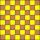How many ways can select 4 fields on a classic chessboard with 64 fields so that fields don't have the same color?
• Triangular landJana has a rectangular garden measuring 30 meters by 72 meters that she wants to split diagonally from corner to corner using a fence. How long does her fence need to be?
• GardenRectangular garden 31 meters 40 centimeters long and 20m30cm wide adjacent with the shorter side with another (fenced) garden and on one longest side is 1 meter wide gate. How many meters of fence is needed to buy? (Estimate, calculate, verify a test calc
• Rectangle - area, perimeterThe area of a rectangular field is equal to 300 square meters. Its perimeter is equal to 70 meters. Find the length and width of this rectangle.
• Playground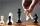On the special playground, there are 81 square sectors, each with a side of 5 m. How many players can fit on the playground if each player needs a 75 m2 area to play?
• HectaresThe rectangular land is 350m long and 200m wide. Calculate the area in hectares.